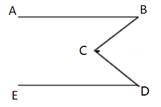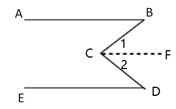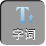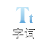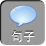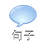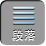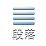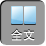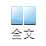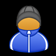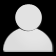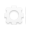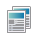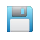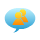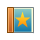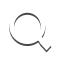-AA+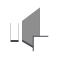“平行线”的特征

1.如图，∠ 1=∠ 2DE// BC，则：① FG// DC；②∠ AED=∠ ACB；③ CD平分∠ ACB；④∠ 1+∠ B= 90°；⑤∠ BFG=∠ BDC；其中正确的结论是（）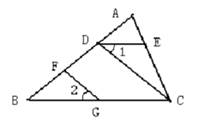A. ①②⑤

B. ①②③

C. ①③④

D. ③④

③错误；

④错误；

1.如图所示， AB// CDOE平分∠ BOCOFOEOPCD，∠ ABO= 40°.则下列结论：①∠ BOE= 70°；② OF平分∠ BOD；③∠ POE=∠ BOF；④∠ POB= 2DOF.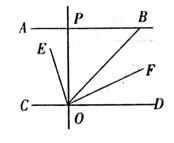A. ①②③④

B. ①②③

C. ①③④

D. ②④

B.

2、如图，已知： EF分别是 ABCD上的点， DEAF分别交 BCGH

A=∠ D，∠ 1=∠ 2，求证：∠ B=∠ C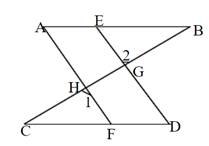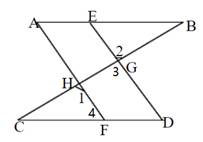∵∠ 1=∠ 2，∠ 3=∠ 2

∴∠ 1=∠ 3

AF// ED

∴∠ 4=∠ D

∵∠ D=∠ A

∴∠ A=∠ 4

AB// CD

∴∠ B=∠ C

2、如图，∠ AED=∠ C，∠ 3=∠ B，求证：∠ 1+∠ 2= 180°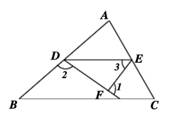∵∠ AED=∠ C

DE// BC

∴∠ EDB+∠ B= 180°

∵∠ 1是△ DEF的外角

∴∠ 1=∠ 3+∠ 4

∵∠ EDB=∠ 2+∠ 4

∴∠ 2+∠ 4+∠ 3= 180°

∴∠ 1+∠ 2= 180°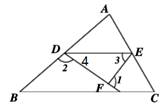3、已知：如图， CDABDE// BCDF// ACFGAB，∠ 1=∠ 2.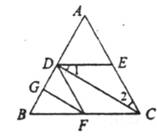CD// GF

∴∠ 3=∠ 6，∠ 4=∠ 5

DE// BC

∴∠ 1=∠ 3

DF// EC

∴∠ 2=∠ 4

∵∠ 1=∠ 2

∴∠ 5=∠ 6

FG平分∠ BFD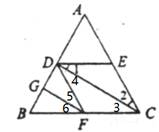3、已知，如图， AB// ED，探究∠ B、∠ D、∠ C的关系.